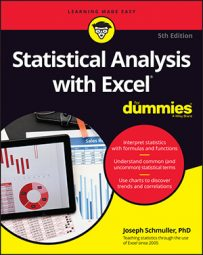##### Statistical Analysis with Excel For DummiesExcel 2016b has a variety of functions for statistical analysis. The Function Library area of the Formulas tab shows all categories of Excel worksheet functions. The steps in using a worksheet function are
1. Type your data into a data array and select a cell for the result.
2. Select the appropriate formula category and choose a function from its pop-up menu. Doing this opens the Function Arguments dialog box.
3. In the Function Arguments dialog box, type the appropriate values for the function’s arguments. Argument is a term from mathematics. It has nothing to do with debates, fights, or confrontations. In mathematics, an argument is a value on which a function does its work.
4. Click OK to put the result into the selected cell.
Yes, that’s all there is to it.

To give you an example, let’s explore a function that typifies how Excel’s worksheet functions work. This function, `SUM`, adds up the numbers in cells you specify and returns the sum in still another cell that you specify. Although adding numbers together is an integral part of statistical number-crunching, `SUM` is not in the Statistical category. It is, however, a typical worksheet function, and it shows a familiar operation.

Here, step by step, is how to use `SUM`:

1. Enter your numbers into an array of cells and select a cell for the result. In this example, enter 45, 33, 18, 37, 32, 46, and 39 into cells C2 through C8, respectively, and selected C9 to hold the sum.
2. Select the appropriate formula category and choose the function from its pop-up menu. This step opens the Function Arguments dialog box. Select `Formulas | Math & Trig` and scroll down to find and choose `SUM`.
3. In the Function Arguments dialog box, enter the appropriate values for the arguments. Excel guesses that you want to sum the numbers in cells C2 through C8 and identifies that array in the Number1 box. Excel doesn’t keep you in suspense: The Function Arguments dialog box shows the result of applying the function. In this example, the sum of the numbers in the array is 250.
4. Click OK to put the sum into the selected cell.
Note a couple of points. First, the Formula bar holds

`=SUM(C2:C8)`

This formula indicates that the value in the selected cell equals the sum of the numbers in cells C2 through C8.

After you get familiar with a worksheet function and its arguments, you can bypass the menu and type the function directly into the cell or into the Formula bar, beginning with an equal sign (=). When you do, Excel opens a helpful menu as you type the formula The menu shows possible formulas beginning with the letter(s) you type, and you can select one by double-clicking it.

Another noteworthy point is the set of boxes in the Function Arguments dialog box. You see just two boxes: Number1 and Number2. The data array appears in Number1. So what's Number2 for?

The Number2 box allows you to include an additional argument in the sum. And it doesn’t end there. Click in the Number2 box, and the Number3 box appears. Click in the Number3 box, and the Number4 box appears — and on and on. The limit is 255 boxes, with each box corresponding to an argument.

A value can be another array of cells anywhere in the worksheet, a number, an arithmetic expression that evaluates to a number, a cell ID, or a name that you have attached to a range of cells. you type values, the `SUM` dialog box shows the updated sum. Clicking OK puts the updated sum into the selected cell.

You won't find this multiargument capability on every worksheet function. Some are designed to work with just one argument. For the ones that work with multiple arguments, however, you can incorporate data that reside all over the worksheet. Here’s a worksheet with a Function Arguments dialog box that includes data from two arrays of cells, two arithmetic expressions, and one cell. Notice the format of the function in the Formula bar — a comma separates successive arguments.

If you select a cell in the same column as your data and just below the last data cell, Excel correctly guesses the data array that you want to work on. Excel doesn’t always guess what you want to do with that array, however. Sometimes when Excel does guess, its guess is incorrect. When either of those things happens, it’s up to you to enter the appropriate values into the Function Arguments dialog box.